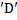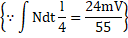# A rodof lengthand massis kept on a horizontal smooth plane. It is free to rotate and move. A particle of same massmoving on the plane with velocitystrikes the rod at pointmaking anglewith the rod. The collision is elastic. After collision,a) The angular velocity of the rod will be 72/55b) The center of the rod will travel a distancein the time in which it makes half rotation c) Impulse of the impact force isd) None of these

## Question ID - 100663 :- A rodof lengthand massis kept on a horizontal smooth plane. It is free to rotate and move. A particle of same massmoving on the plane with velocitystrikes the rod at pointmaking anglewith the rod. The collision is elastic. After collision,a) The angular velocity of the rod will be 72/55b) The center of the rod will travel a distancein the time in which it makes half rotation c) Impulse of the impact force isd) None of these

3537

(a,b,c)

The ball hascomponent of its velocity perpendicular to the length of the rod immediately after collision.is the velocity of CM of the rod andis angular velocity of the rod just after collision. The ball strikes the rod with a speed ofin the perpendicular direction and its component along the length of the after the collision is unchangedUsing for the point of collision

Velocity of separation= Velocity of approach…(i)

Conserving linear momentum (of rod+ particle) in the direction perpendicular to the rod…(ii)

Conserving angular momentum about pointas shown in the figureTime taken rotate byangle,In the same time, distance travelled =Using angular impulse- angular momentum equation[Using impulse-momentum equation on the rodNext Question :

In the following question letters/letter groups are arranged in a particular order with some underlying criterion.  Study the order and choose the answer from the alternative to fill the gaps.

 DKY, FJW, HIU, JHS, ……… (a) KGR (b) KFR (c) KFS (d) LGQ